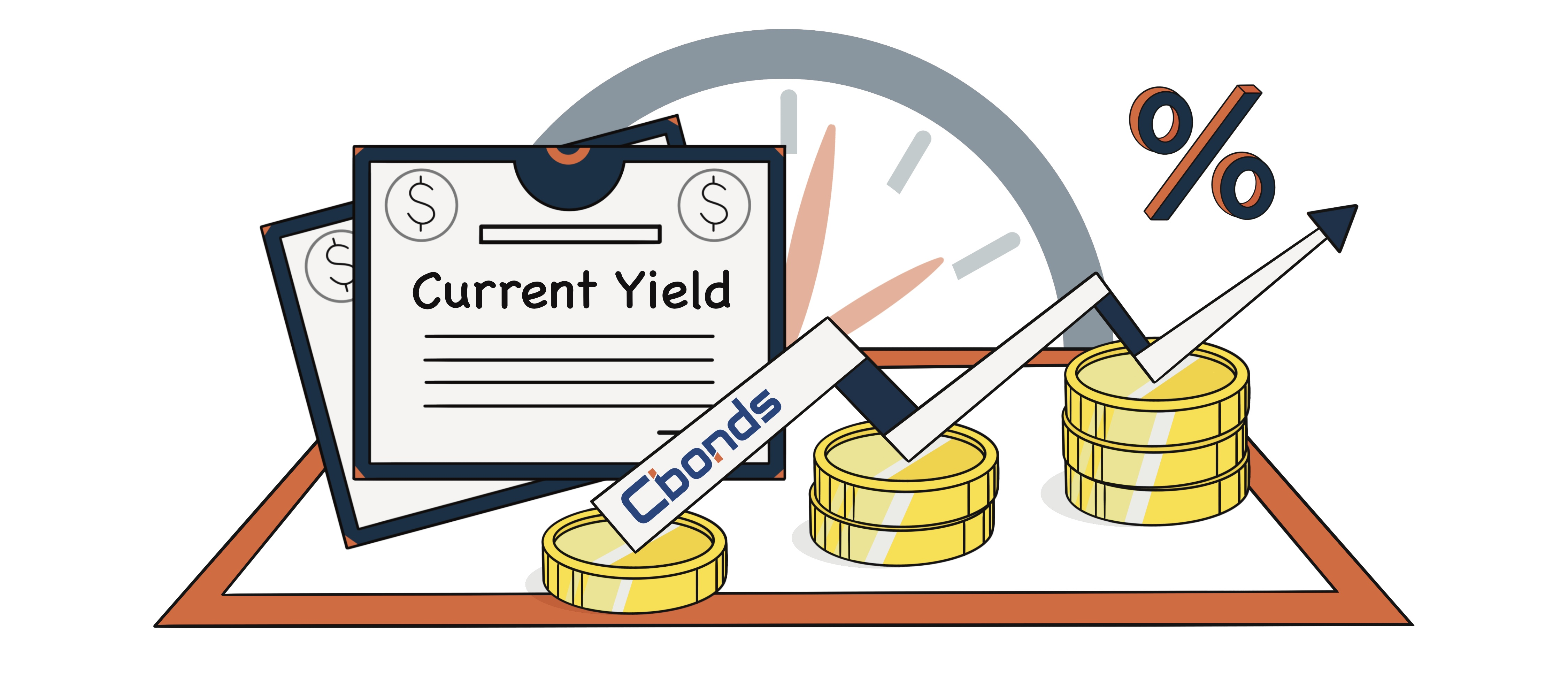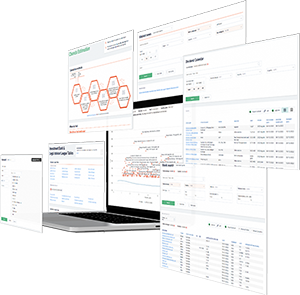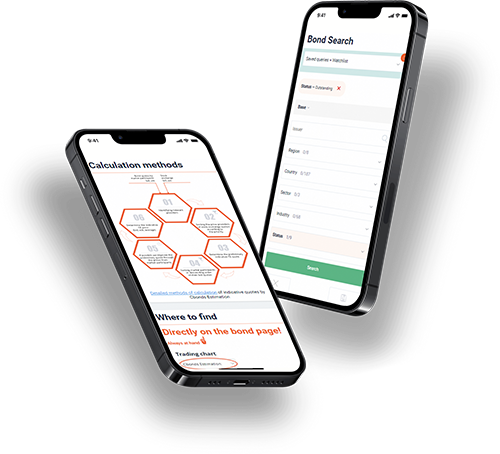Hint mode is switched on
Glossary

# Current Yield

Category — Analytical Metrics

## What Is the Current Yield?

The current yield of an investment is the annual income (interest or dividends) divided by the security’s current price. This metric focuses on the current price of a bond rather than its face value. It represents the expected return for an investor who purchases the bond and holds it for a year. However, it does not reflect the actual return an investor would receive if they hold the bond until maturity.

To calculate the current yield, divide the annual income by the bond’s current market price. It is important to note that the current yield formula expresses the yield as a percentage. The current yield provides insight into the return an investor can expect from their bond investment based on the bond’s current market price.## Understanding Current Yield

The concept of current yield is commonly applied to bond investments. Bonds are financial securities issued to investors with a par value or face amount typically set at \$1,000. These bonds carry a stated coupon rate of interest, which is indicated on the bond certificate. Investors engage in trading bonds, and the market price of a bond can fluctuate. As a result, an investor may purchase a bond either at a discount (below par value) or at a premium (above par value). The purchase price of a bond has an impact on its current yield.

To calculate the current yield, you can utilize the annual coupon payment formula divided by the bond’s current market price. The current yield is typically expressed as a percentage. It provides valuable information to investors regarding the potential return a bond investment generates based on its current market price.

In summary, the current yield considers factors such as the coupon rate, par value, premium or discount bond pricing, and the current market prices of bonds. It helps investors determine the relative attractiveness of different bonds and aids in making informed investment decisions.

## How to Calculate Current Yield?

The current yield formula can be expressed as follows:

Current yield = Annual coupon payment / Current bond price

To calculate the current yield, you need to obtain the annual coupon payment, which is the fixed interest amount stated on the bond certificate. Additionally, it would be best to determine the bond’s current price, reflecting its trading value in the market. The bond may be trading at par, meaning its market price is equal to its face value. Alternatively, the bond may be trading at a premium, indicating a market price higher than the face value or at a discount, representing a market price lower than the face value.

## Current Yield Calculation Example

Suppose an investor purchases a bond with a 6% coupon rate at a discounted price of \$900. In this scenario, the investor would earn an annual interest income of (\$1,000 x 6%), which amounts to \$60. Calculating the current yield involves dividing the annual interest of \$60 by the purchase price of \$900, resulting in a current yield of 6.67%. It’s important to note that the \$60 annual interest remains fixed, regardless of the bond’s price.

On the other hand, if the investor acquires a bond at a premium price of \$1,100, the current yield would be calculated as (\$60) / (\$1,100), giving a current yield of 5.45%. In this case, the investor paid more for the premium bond, even though it generates the same dollar amount of interest. As a result, the current yield is lower due to the higher purchase price.

Additionally, current yield can be computed for stocks by dividing the dividends received for a stock by its current market price.

In summary, the current yield is a measure used to assess the return on investment for bonds and stocks. It is calculated by dividing the annual income (such as interest or dividends) by the respective security’s market price. By considering current yield, investors can compare different investment options and evaluate their relative value.

## Current Yield vs. Yield to Maturity

The current yield and yield-to-maturity (YTM) formulas are two distinct methods utilized to calculate a bond’s yield. However, their applications vary depending on the specific goals of an investor.

Yield to maturity (YTM) represents the overall return an investor can expect to achieve by holding a bond until its maturity date. It takes into account both the periodic interest payments and the principal repayment at maturity. Let’s consider an example to illustrate this concept.

Suppose an investor purchases a bond with a coupon rate of 6% at a discount price of \$900. The bond has a maturity period of 10 years. To calculate the YTM, the investor needs to estimate a discount rate that discounts the future cash flows (interest payments and the principal repayment) to their present value.

In this particular case, the investor receives \$60 in annual interest payments for 10 years. Upon maturity, the investor will receive the bond’s par value of \$1,000, resulting in a \$100 capital gain. By summing up the present value of the interest payments and the capital gain, the YTM of the bond can be calculated. If the bond is purchased at a premium, the YTM calculation would include a capital loss since the bond matures at par value.

In summary, the YTM provides a comprehensive measure of the total return on a bond investment, accounting for both interest payments and any capital gains or losses realized upon maturity. It is derived by discounting future cash flows back to their present value using an assumed discount rate.

## What the Current Yield of a Bond Can Tell You

The current yield of a bond is determined by dividing its annual coupon payment by the bond’s current market value. This calculation provides a more accurate assessment of the bond’s profitability compared to its market peers, as it is based on the purchase price rather than the par value. The current yield calculation is particularly useful for short-term investments, as it helps investors identify bonds that offer higher annual returns.

For instance, let’s consider an investor who purchases a bond with a 6% coupon rate and a par value of \$1,000 for a discounted price of \$900. In this case, the investor receives an annual interest income of (\$1,000 X 6%), which amounts to \$60. By dividing this annual interest payment by the bond’s purchase price of \$900, the current yield is determined to be 6.67%. It is important to note that the \$60 in annual interest remains constant regardless of the price paid for the bond.

Conversely, if the investor buys a bond at a premium price of \$1,100, the current yield would be calculated as (\$60) / (\$1,100), resulting in a lower current yield of 5.45%. This is because the investor paid a higher price for the premium bond that generates the same dollar amount of interest.

In summary, the current yield is a valuable metric for evaluating the annual returns on bond investments. By considering the bond’s coupon payment and market value, investors can assess the relative profitability of different bonds in the market.

Get access
×

## — We have everything you need:full data on over 700 000 bonds, stocks & ETFs; powerful bond screener; over 350 pricing sources among stock exchanges & OTC market; ratings & financial reports; user-friendly interface; available anywhere via Website, Excel Add-in and Mobile app.

×

## WhyYou will have detailed descriptive & pricing data for 650K bonds, 76K stocks, 8K ETFsGet full access to the platform from any device & via Cbonds app